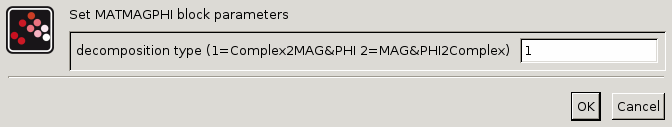Scilab Home page | Wiki | Bug tracker | Forge | Mailing list archives | ATOMS | File exchange
Change language to: English - Français - Português - 日本語 -
Справка Scilab >> Xcos > palettes > Math operations palette > MATMAGPHI

# MATMAGPHI

Complex from/to Magnitude and Angle Conversion

### Block Screenshot### Description

This block computes the magnitude and the angle of a matrix of complex numbers or composes a matrix of complex numbers from a matrix of magnitudes and a matrix of angles with respect to the value of decomposition type parameter. The following table summarize the bloc behaviour :

 Decomposition type Inputs Outputs Operation 1 One (complex or double) Two (double) Conversion complex to magnitude (first output) and radian angle (second output). If the input is double, the angle will be zero or PI and the magnitude will be equal to the absolute of the input number. 2 Two (double) One (complex) The block outputs a matrix of complex numbers built with the magnitude and the radian angle inputs coming respectively from the first and from the second inputs.

### Parameters• Decomposition type (1=Complex2MAG&PHI 2=MAG&PHI2Complex)

It indicates the rule of the conversion.

Properties : Type 'vec' of size 1.

### Default properties

• always active: no

• direct-feedthrough: yes

• zero-crossing: no

• mode: no

• regular inputs:

- port 1 : size [-1,-2] / type 2

• regular outputs:

- port 1 : size [-1,-2] / type 1

- port 2 : size [-1,-2] / type 1

• number/sizes of activation inputs: 0

• number/sizes of activation outputs: 0

• continuous-time state: no

• discrete-time state: no

• object discrete-time state: no

• name of computational function: matz_abs

### Interfacing function

• SCI/modules/scicos_blocks/macros/MatrixOp/MATMAGPHI.sci

### Computational function

• SCI/modules/scicos_blocks/src/c/matz_abs.c

• SCI/modules/scicos_blocks/src/c/matz_absc.c